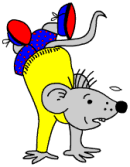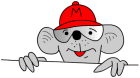## Free Y5 maths worksheets: Revise understanding subtraction

Subtraction is non-commutative.
When a larger number is subtracted from a smaller number, the answer is negative.Two free maths worksheets, very similar to the year 4 ones on saying whether statements are true or false, with slightly harder numbers. This is revision of the following concepts:

Subtraction is the same as taking away, finding the difference between, and complementary addition.

Subtraction is non-commutative.
When a larger number is subtracted from a smaller number, the answer is negative.

Subtracting a number from another makes it smaller.
Subtracting zero makes no difference to a number.

Subtraction is the inverse of addition.

They should have good mental strategies for solving subtraction problems with simple numbers.

Free Y5 maths worksheets: Revise understanding subtraction (pg 2)

## Free Y4 maths worksheets: Understand subtractionBy year 4 expectations are rising as children’s understanding and knowledge increases. The Primary Framework for Mathematics suggests that children should be able to read, write and understand the following words:

Take away

subtract

how many are left?

how much less?

difference between,

how much more?

how many more to make?

decrease,

inverse

and the minus sign (–)

They should know that:

Subtraction is the same as taking away, finding the difference between and complementary addition.

Subtraction is non-commutative.

When a larger number is subtracted from a smaller number, the answer is negative.

Subtracting a number from another makes it smaller.

Subtracting zero makes no difference to a number.

Subtraction is the inverse of addition.

They should have good mental strategies for solving subtraction problems with simple numbers.

These two free maths worksheets can be used as a check to see whether some of these fundamentals have been understood.

Free Y4 maths worksheets: Understand subtraction and its relationship to addition (pg 2)

On the same day that I post my first reading book to help young children with counting this news comes from the BBC.

“The reading performance of children in England has fallen from third to 19th in the world in a major assessment. The Progress in International Reading Literacy Study (Pirls), undertaken every five years, involved children aged about 10 in 40 countries. Scotland also fell, from 14th to 26th. Russia, which matched it last time, was top of the overall achievement table.” Continue reading “News: Reading performance slumps!”

## Free Y3 maths worksheets: Understand subtractionThe work on subtraction facts in year 3 is very similar to that in year 2. Constant practice and re-inforcement is necessary if children are going to become quick in their responses – the numbers do not necessarily have to be larger, but a more rapid answer should be expected.

A similar list of words and phrases should be used as for year 2, including:

take away, subtract, how many are left, less than,
what is the difference between, more than, how many more to make ….

It is expected that the minus (–) sign can be read and written.

Most of the work will still be done orally. All kinds of opportunities arise where simple subtraction questions can be posed, but the numbers do need to be kept simple.

## Free Y2 maths worksheets: Take whole tens

a number line is really useful for helping children gain confidence with subtraction.Here we have our first two free maths worksheets on subtraction for Year 2. Both these pages are about subtracting whole tens, with a number line to help. As stated in the Year 1 subtraction worksheets, a number line is really useful for helping children gain confidence with subtraction.

Make sure that they are competent and confident with counting up and down in tens, up to 100, before trying these, as success depends on this ability.

Free Y2 maths worksheets: Take whole tens (pg 1)

Free Y2 maths worksheets: Take whole tens (pg 2)

## Free Y1 maths worksheets: Understand subtraction

take away, subtract, how many are left, less than, what is the difference between, more than.Much of the basic vocabulary involved with subtraction is introduced in year 1, including these terms:

take away, subtract, how many are left, less than, what is the difference between, more than.

The minus (-) sign is also introduced.

As with addition, at this age most of the work will be done orally. All kinds of opportunities arise where simple subtraction questions can be posed.

## Free Y6 maths worksheets: Multi-step operationsJust one maths worksheet with just 8 questions, but be warned this might prove to be tricky for most 10 or 11 years old children. At least two operations are needed to find the answer, and as I have said before this causes most children a fair amount or difficulty. This is where logical thinking comes in: what to do first in order to make it possible to move on to the second step.

Free Y6 maths worksheets: Multi-step operations (pg 5)

## Free Y5 maths worksheets: Choose the operation and methodVery similar to the year 4 worksheets on the same subject, this re-inforces and emphasises the importance of being able to explain the methods used to reach an answer. In the ‘Operation’ box the child should put whether to add, subtract, multiply or divide. The ‘Method’ refers to whether it was done mentally, with paper and pencil or with a calculator. The ‘How’ should give more detail as to exactly how the question was carried out: what maths was actually done to reach the answer.

Free Y5 maths worksheets: Choose the operation and method (pg 6)

## Free Y4 maths worksheets: Choose the operation and method

Which operation is involved in word problems
Whether calculations should be done mentally or with pen and paper.
How to explain and record the operations used in solving the problem.These two maths worksheets are concerned with getting children to make decisions about:

Which operation is involved in word problems

Whether calculations should be done mentally or with pen and paper.

How to explain and record the operations used in solving the problem.

They focus on examples and draw children’s attention to these issues, but it is important to realise that these decisions should be taken by children in most things they do in mathematics, so encourage them to discuss their work wherever possible. Often there is no one right way to tackle a problem, but usually there is a most efficient way which is what they should be looking for.

Free Y4 maths worksheets: Choose the operation and method (pg 6)

## Maths game: Counting mice (counting up to 5)

The mice are escaping with the cheese? How many can you see?

The final maths game (for the moment) in our series of counting up to 5.